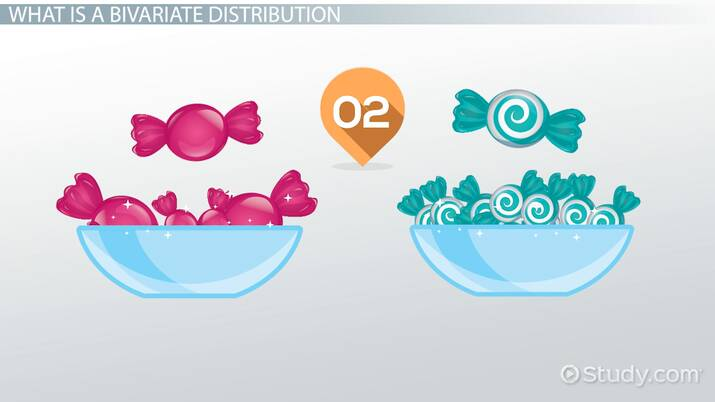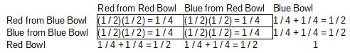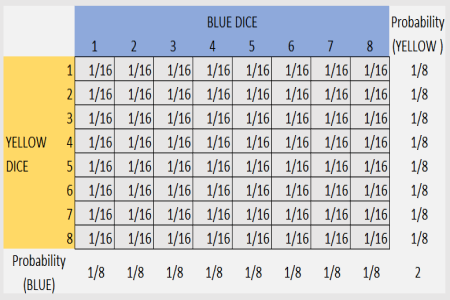# Bivariate Distributions: Definition & Examples

Lesson Transcript
Instructor
Yuanxin (Amy) Yang Alcocer

Amy has a master's degree in secondary education and has been teaching math for over 9 years. Amy has worked with students at all levels from those with special needs to those that are gifted.

Expert Contributor
Christianlly Cena

Christianlly has taught college physics and facilitated laboratory courses. He has a master's degree in Physics and is pursuing his doctorate study.

A bivariate distribution shows the joint probabilities of two random variables. Explore the definition of bivariate distribution and discover what a bivariate distribution looks like through the given examples. Updated: 01/25/2022

## What Is a Bivariate Distribution

A bivariate distribution, put simply, is the probability that a certain event will occur when there are two independent random variables in your scenario. For example, having two bowls, each filled with two different types of candies, and pulling one candy from each bowl gives you two independent random variables: the two different candies.

Since you're pulling one candy from each bowl at the same time, you have a bivariate distribution when calculating your probability of ending up with particular kinds of candies.An error occurred trying to load this video.

Try refreshing the page, or contact customer support.

Coming up next: Marginal & Conditional Probability Distributions: Definition & Examples

### You're on a roll. Keep up the good work!

Replay
Your next lesson will play in 10 seconds
• 0:04 What Is a Bivariate…
• 0:36 What Does it Look Like?
• 1:15 Digging Deeper
• 2:07 Example
• 2:42 Lesson Summary
Save Save

Want to watch this again later?

Timeline
Autoplay
Autoplay
Speed Speed

## What Does it Look Like?

So what does a bivariate distribution look like? Such a distribution actually doesn't have a standard look. You can create a table with these distributions or you can list each probability out one by one. In any case, there are always two independent random variables in any given scenario.

Here is what a bivariate distribution looks like in table form:This bivariate distribution shows you the probability of picking red or blue candies from a red bowl and a blue bowl if you pick one candy from each bowl, and there are an equal number of red and blue candies in each bowl.

## Digging Deeper

Once you have your distribution, you can look at the numbers to see what your probabilities are for each possible option in your scenario. Looking at the table for the candies again, you see four options (two red candies, two candies from opposite colored bowls, two candies from same colored bowls, and two blue candies). The option for getting two red candies has a probability of one in four.

To unlock this lesson you must be a Study.com Member.

## Bivariate Distributions: True or False Activity

This activity will help assess your knowledge regarding the meaning and examples of a bivariate distribution.

Guidelines

Print or copy this page on a blank piece of paper. Based on the given scenario, identify whether the following statement is TRUE or FALSE. Neatly write your answers in the appropriate space provided.

Blake tosses a pair of fair, eight-sided dice where one of the dice is BLUE, and the other is YELLOW. Below is a table displaying the probability of obtaining a pair of numbers after Blake rolls the two dice. Additionally, it also shows the probability of obtaining a number after rolling one dice.__________ 1. All probabilities listed in the table are correct.

__________ 2. The scenario above is an example of a bivariate distribution.

__________ 3. The sum of probabilities in rolling the blue dice should be equal to 2.

__________ 4. The sum of the probabilities in rolling the yellow dice should be equal to 1.

__________ 5. The probability of rolling (2,3) with the two dice is 1/48.

__________ 6. Rolling a 7 with the yellow dice has a chance of 1/8.

__________ 7. The two independent variables are the two numbers obtained from rolling two dice at the same time.

__________ 8. The probability of rolling the two dice to get a total of 8 is 8/64.

1. FALSE, because the correct statement is: Some probabilities listed in the table are incorrect.

2. TRUE

3. FALSE, because the correct statement is: The sum of probabilities in rolling the blue dice should be equal to 1.

4. TRUE

5. FALSE, because the correct statement is: The probability of rolling (2,3) with the two dice is 1/64.

6. TRUE

7. TRUE

8. FALSE, because the correct statement is: The probability of rolling the two dice to get a total of 8 is 7/64.

### Register to view this lesson

Are you a student or a teacher?